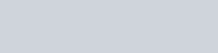Magnetic Effect of Electric Current Class 12 Notes | Introduction
The electricity and magnetism are linked to each other and it is proved when the electric current passes through the copper wire, it produces a magnetic effect. The electromagnetic effects first time noticed by Hans Christian Oersted. Oersted discovered a magnetic field around a conductor carrying an electric current. The magnetic field is a quantity, which has both magnitude and direction. The direction of a magnetic field is usually taken to be the direction in which, a north pole of the compass needle moves inside it. So here you will get Magnetic Effect of Electric Current Class 12 complete Notes to prepare for Boards as well as for JEE & NEET Exams.Oersted discovered a magnetic field around a conductor carrying an electric current. Other related facts are as follows:
(a) A magnet at rest produces a magnetic field around it while an electric charge at rest produces an electric field around it.
(b) A current-carrying conductor has a magnetic field and not an electric field around it. On the other hand, a charge moving with a uniform velocity has an electric as well as a magnetic field around it.
(c) An electric field cannot be produced without a charge whereas a magnetic field can be produced without a magnet.
(d) No poles are produced in a coil carrying current but such a coil shows north and south polarities.
(e) All oscillating or an accelerated charge produces E.M. waves also in addition to electric and magnetic fields.### Unit of Magnetic field

UNIT OF $\overrightarrow{\mathrm{B}}:$ MKS weber/metre $^{2},$ SI tesla, CGS maxwell cm’ or gauss.

One Tesla $=$ one (weber/m’) $=10^{4}$ (maxwell/cm’) $=10^{4}$ gauss

## Biot-Savart’s Law

With the help of experimental results, Biot and Savart arrived at a mathematical expression that gives the magnetic field at some point in space in terms of the current that produces the field. That expression is based on the following experimental observations for the magnetic field $\overrightarrow{\mathrm{d} B}$ at a point $P$ associated with a length element $\overrightarrow{\mathrm{d} \ell}$ of a wire carrying a steady current I.$\mu_{0}$ is called permeability of free space $\frac{\mu_{0}}{4 \pi}=10^{-7}$ henry/meter.

$1(\mathrm{H} / \mathrm{m})=1 \frac{\mathrm{T} \mathrm{m}}{\mathrm{A}}=1 \frac{\mathrm{Wb}}{\mathrm{Am}}=1 \frac{\mathrm{N}}{\mathrm{A}^{2}}=1 \frac{\mathrm{Ns}^{2}}{\mathrm{c}^{2}}$

DIMENSIONS of $\mu_{0}=\left[\mathrm{M}^{\prime} \mathrm{L}^{\prime} \mathrm{T}^{-2} \mathrm{A}^{-2}\right]$

For vaccum $: \sqrt{\frac{1}{\mu_{0} \varepsilon_{0}}}=\mathrm{c}=3 \times 10^{8} \mathrm{m} / \mathrm{s}$

### Biot-Savart law in Vector form[Note: Static charge is a source of electric field but not of magnetic field, whereas the moving charge is a source of electric field as well as magnetic field.]

the direction of $\mathrm{d} \mathrm{B}$ is perpendicular to the plane determined by $\overrightarrow{\mathrm{d} \ell}$ and $\overrightarrow{\mathrm{r}}$ (i.e. if $\overrightarrow{\mathrm{d} \ell}$ and $\overrightarrow{\mathrm{r}}$ lie in the plane of the paper then $\overrightarrow{\mathrm{dB}}$ is $\perp$ to plane of the paper). In the figure, direction of

$\overrightarrow{\mathrm{dB}}$ is into the page. (Use right hand screw rule).